Courses

# NCERT Solutions(Part- 3)- Comparing Quantities Class 8 Notes | EduRev

## Class 8 Mathematics by VP Classes

Created by: Full Circle

## Class 8 : NCERT Solutions(Part- 3)- Comparing Quantities Class 8 Notes | EduRev

The document NCERT Solutions(Part- 3)- Comparing Quantities Class 8 Notes | EduRev is a part of the Class 8 Course Class 8 Mathematics by VP Classes.
All you need of Class 8 at this link: Class 8

Exercise 8.2

Question 1. A man got a 10% increase in his salary. If his new salary is Rs 1,54,000, find his original salary.

Solution: Let the original salary = Rs x

∴ Increase in salary = 10% of Rs x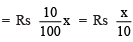New salary =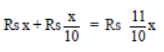∴ Rs 11/10x = Rs 154000

or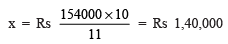Thus, the original salary = Rs 1,40,000

Question 2. On Sunday 845 people went to the Zoo. On Monday only 169 people went. What is the per cent decrease in the people visiting the Zoo on Monday?

Solution:

Number of visitors on Sunday = 845

Number of visitors on Monday = 169

Decrease in the number of visitors = 845 – 169 = 676

∴ Per cent decrease =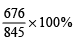= (4 * 20)% = 80%

Question 3. A shopkeeper buys 80 articles for Rs 2,400 and sells them for a profit of 16%. Find the selling price of one article.

Solution: Cost price = Rs 2400

Profit = 16% of Rs 2400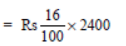= Rs 16 * 24 = Rs 384

∴ Selling price = Rs 2400 + Rs 384 = Rs 2784

Now, selling price per article = Rs 2784/80      [∵ Number of articles = 80]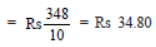Question 4. The cost of an article was Rs 15,500. Rs 450 were spent on its repairs. If it is sold for a profit of 15%, find the selling price of the article.

Solution:

Total cost = Rs 15500 + Rs 450 (Overhead expenses)

= Rs 15950

Profit = 15% of Rs 15950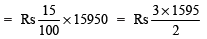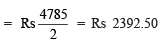∴ Selling price = Rs 15950 + Rs 2392.50

= Rs 18342.50

Question 5. A VCR and TV were bought for Rs 8,000 each. The shopkeeper made a loss of 4% on the VCR and a profit of 8% on the TV. Find the gain or loss per cent on the whole transaction.

Solution:

For VCR                                                                      For TV

CP = Rs 8000                                                         CP = Rs 8000

Loss = 4% of Rs 8000                                          Profit = 8% of Rs 8000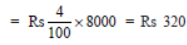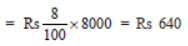∴ SP = Rs 8000 – Rs 320                                   SP = Rs 8000 + Rs 640

Now, total CP = Rs 8000 + Rs 8000 = 16000

Total SP = Rs 7680 + Rs 8640 = Rs 16320

∵ SP > CP

∴ Overall profit = Rs 16320 – Rs 16000 = Rs 320

Overall profit per cent =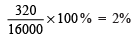Question 6. During a sale, a shop offered a discount of 10% on the marked prices of all the items. What would a customer have to pay for a pair of jeans marked at Rs 1450 and two shirts marked at Rs 850 each?

Solution:

For a pair of Jeans                                  For two Shirts

Marked price = Rs 1450                        Marked price = (Rs 850) x 2 = Rs 1700

Discount = 10% of Rs 1450                   Discount = 10% of Rs 1700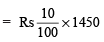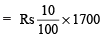= Rs 145                                                 = Rs 170

∴ Sale price = Rs 1450 – Rs 145           ∴ Sale Price = Rs 1700 – 170

= Rs 1305                                               = Rs 1530

Total sale price = Rs 1305 + Rs 1530

= Rs 2835

Thus, the customer would have to pay Rs 2835.

Question 7. A milkman sold two of his buffaloes for Rs 20,000 each. On one he made a gain of 5% and on the other a loss of 10%. Find his overall gain or loss.(Hint: Find CP of each.)

Solution:

For 1st buffalo                                          For 2nd buffalo

SP = Rs 20,000                                        SP = Rs 20,000

Profit = 5% Loss = 10%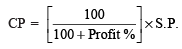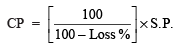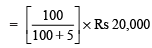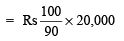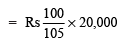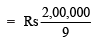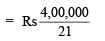Now, Overall SP = Rs 2 * 20,000

= Rs 40,000

Overall CP =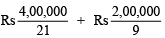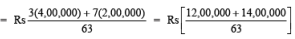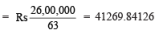= Rs 41269.84

Now, CP > SP

∴ Overall loss = Rs (41269.84 – 40000)

= Rs 1269.84

Question 8. The price of a TV is Rs 13,000. The sales tax charged on it is at the rate of 12%. Find the amount that Vinod will have to pay if he buys it.

Solution:

Cost (sale price) of the TV = Rs 13,000

Rate of sales tax = 12%

∴  Sales tax = 12% of Rs 13000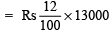= Rs (12 * 130) = Rs 1560

∴  Bill amount = Rs 13000 + Rs 1560

= Rs 14,560

Thus Vinod will have to pay Rs 14,560 for the TV.

Question 9. Arun bought a pair of skates at a sale where the discount given was 20%. If the amount he pays is Rs 1,600, find the marked price.

Solution:

Let the marked price = Rs 100

∴ Discount = 20% of Rs 100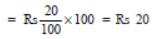Sale price = Rs (100 – 20) = Rs 80

If sale price is Rs 80, then marked price = Rs 100

If sale price is Rs 1600, then marked price =  Rs 100/80 *  1600 = Rs 2000

Question 10. I purchased a hair-dryer for Rs 5,400 including 8% VAT. Find the price before VAT was added.

Solution: Let the original price = Rs 100

∴  Original price + VAT = Rs 100 + Rs (8% of 100)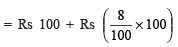= Rs 108

∴ Bill amount = Rs 108

If bill amount is Rs 108, then original price = Rs 100

If bill amount is Rs 5,400, then original price = Rs 100/108 * 5400

= Rs (100 * 50)

= Rs 5000

Compound Interest

Interest is the extra money paid by banks, post offices or people when they borrow money. In simple interest, the principal remains the same each year.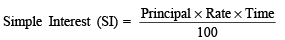Amount = Principal + Interest

Application of Compound Interest Formula

The formula A =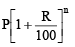can use to find:

(i) Increase (or decrease) in population.

(ii) The growth of a bacteria if the rate of growth is known.

(iii) The value of an item, if its price increase or decrease in the intermediate years.

Example 1. A car was purchased for Rs 2,10,000. After 2 years the value of the car depreciated 5%. Find the value of the car after one year.

Note: Depreciation means reduction of value due to use and age of the item.

Solution: We have P = Rs 2,10,000, R = 5% p.a., T = 2 years

∵ Reduction is there, we use the following formula:

∴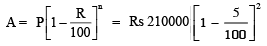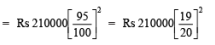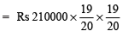= Rs (525 * 19 * 19) = Rs 1,89,525

∴ The value of the car after 2 years = Rs 1,89,525

Question: Find interest and amount to be paid on Rs 15000 at 5% per annum after 2 years.

Solution: Here, Principal = Rs 15000

Rate of interest = 5% per annum

Time = 2 years

∵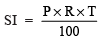∴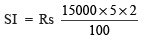= Rs (150 * 5 * 2) = Rs 1500

∵ Amount = P + SI

∴ Amount = Rs 15000 + Rs 1500 = Rs 16500

Note:

I. When interest is calculated on the amount of previous year then it is called compound interest (CI).

II. Unlike simple interest, the formula for compound interest, i.e.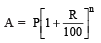gives us the amount directly.

Offer running on EduRev: Apply code STAYHOME200 to get INR 200 off on our premium plan EduRev Infinity!

93 docs|16 tests

,

,

,

,

,

,

,

,

,

,

,

,

,

,

,

,

,

,

,

,

,

;